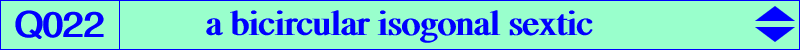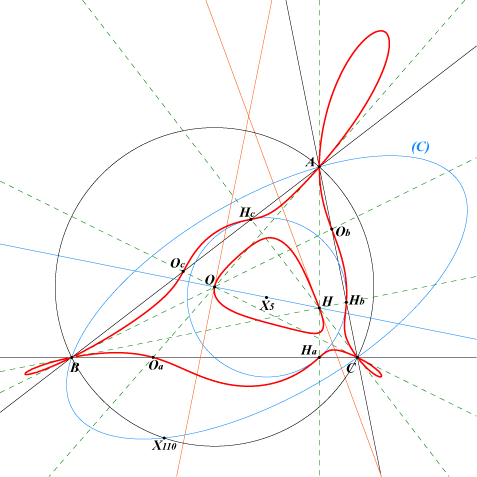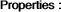too complicated to be written here. Click on the link to download a text file.X(3), X(4) points at infinity of the circum-conic (C) with center X(5), perspector X(216) Ha, Hb, Hc : vertices of the orthic triangle Oa, Ob, Oc : vertices of the cevian triangle of OQ022 is a bicircular isogonal sextic. It is the locus of P such that the orthotransversals of P and its isogonal conjugate P* are perpendicular (together with the line at infinity and the circumcircle). See also Q021.A, B, C are double points. The tangents at A are the line AO and AH. The tangent at O is perpendicular to the Euler line. The tangent at H passes through X(924). Q022 is tritangent at Ha, Hb, Hc to the nine-point circle.